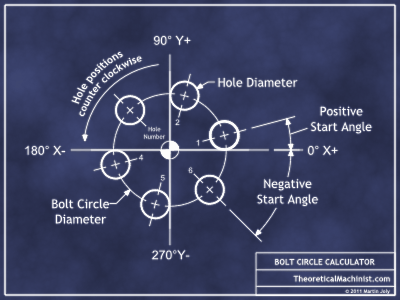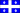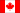# Bolt Hole Circle Calculator

Dimensions
Bolt circle diameter:
Hole diameter:
Number of holes:
Start angle:
Output Unit Precision
1 2 3 4 5

Locations
NC format listing
Hole Locations
position data

### Glossary

Units of measure:
The units of measure used when defining a bolt circle hole list must all be of the same type, for example: all in inches or all in millimetres.
Hole diameter:
The diameter of the holes that are equally distributed around the bolt circle diameter
Bolt circle diameter:
the diameter of the circle on which the holes will be evenly distributed.
Start angle:
The 0° angle is to the right in the "X" axis and aligned with the center of the bolt circle in the "Y" axis. The angle is described as...
- A positive angle is counterclockwise
- A negative angle is clockwise. ex:-45° = 315°
Angle units
360° = 21,600 minutes = 1,296,000 seconds = 6.2832 radians = full circle
(1 degree) = 60 minutes = 3,600 seconds = 0.0175 radian
0°1' (1 minute of a degree) = .016 degree = 60 seconds = .000298 radian
0°0'1" (1 second of a degree) = .00027 degree = .0000048 radian
Angle entry field (Convert decimal angle to; degrees, minutes and seconds)
Angle format example 1: decimal 15.5° enter as 15.5 no formating needed.
Angle format example 2: 64°45'34" enter as 64d45m34s
Angle format example 3: -30°5' enter as -30d5m
Angle format example 4: 15°0'10" enter as 15d10s
Angle format example 5: -0°0'50" enter as -50sLast Updated: 12 April 2015

Decimal Degree Sexagesimal Degree
 Privacy policy |  Disclaimer |  TheoreticalMachinist.com Copyright © 2011-2018 Joly Concept